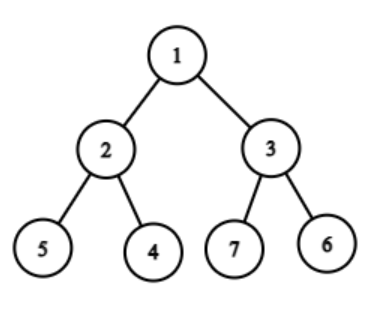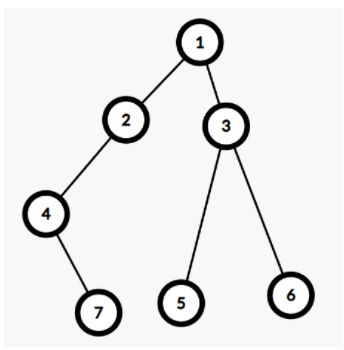Posted: 2 Dec, 2020
Difficulty: Easy

## PROBLEM STATEMENT

#### A binary tree is a hierarchical data structure in which each node has at most two children.

##### Example:
``````Consider the given binary tree:
````````````For the given tree the leaf nodes are 5, 4, 7, 6. In this binary tree, the maximum sum path is 11(1 + 3 + 7). So the path 1 3 7 is printed.
``````
##### Input format :
``````The first line contains a single integer T representing the number of test cases.

The first line of each test case contains elements in the level order form(refer to the example below) (separated by space). If any node does not have a left or right child, take -1 in its place.
``````
##### Example:
``````Elements are in the level order form. The input consists of values of nodes separated by a single space in a single line. In case a node is null, we take -1 in its place.

For example, the input for the tree depicted in the below image would be :
1
2 3
4 -1 5 6
-1 7 -1 -1 -1 -1
-1 -1
````````````Explanation :
Level 1 :
The root node of the tree is 1

Level 2 :
Left child of 1 = 2
Right child of 1 = 3

Level 3 :
Left child of 2 = 4
Right child of 2 = null (-1)
Left child of 3 = 5
Right child of 3 = 6

Level 4 :
Left child of 4 = null (-1)
Right child of 4 = 7
Left child of 5 = null (-1)
Right child of 5 = null (-1)
Left child of 6 = null (-1)
Right child of 6 = null (-1)

Level 5 :
Left child of 7 = null (-1)
Right child of 7 = null (-1)

The first not-null node (of the previous level) is treated as the parent of the first two nodes of the current level. The second not-null node (of the previous level) is treated as the parent node for the next two nodes of the current level and so on.

The input ends when all nodes at the last level are null (-1).

Note: The above format was just to provide clarity on how the input is formed for a given tree.

The sequence will be put together in a single line separated by a single space. Hence, for the above-depicted tree, the input will be given as:

1 2 3 4 -1 5 6 -1 7 -1 -1 -1 -1 -1 -1
``````
##### Output format :
``````For each test case, print "True" if all the leaf nodes are at the same level otherwise print "False” (without quotes).

The output of each test case should be printed in a separate line.
``````
##### Note
``````You are not required to print anything, it has already been taken care of. Just implement the function.
``````
##### Constraints:
``````1 <= T <= 100
1 <= N <= 3000
-10^9 <= nodeVal <= 10^9

Where nodeVal denotes the value associated with the node.

Time Limit: 1 sec
``````Approach 1

We can run a Depth-First Search (DFS) from the root of the tree and calculate the sum path of all leaves from the root. Among all the sum paths of the leaves print the maximum sum path.

Algorithm:-

1. Run a depth-first search (DFS) from node 1.
2. For each node store the sum of the path and the path of the node from the root.
1. If the sum of the path of the parent node is ‘X’ then update the sum of the path of the current node as ‘X ’+value of the current node.
2. To update the path add the current node to the path of the parent node and store the path.
3. Choose the leaf with the maximum sum and print the path from the root to this leaf.